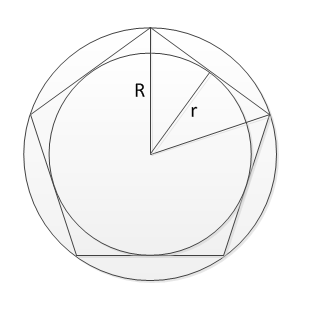# Regular polygon, number of sides and length of side from incircle and circumcircle radii

This online calculator finds number of sides and length of side of regular polygon given the radii of incircle and circumcircleWell, I have to come back to incircles and circumcircles. I thought I've done with them after I've created Side length of the regular polygon to calculate length of polygon and Regular Polygon Incircle and Circumcircle Calculator to calculate radii, but I was wrong.

One of site visitors asked for "calculator which finds length of polygon side from incircle and circumcircle radii".

So, the problem is:
Some regular polygon has incircle with known radius and circumcircle with known radius. You need to find number of sides of this polygon and length of the side.

To make it clear, there is a picture on the left, presenting this situation.

If we look at triangle created by incircle radius r, circumcircle radius R and half side of regular polygon, we can see that there radii related by the formula

There alpha angle related to number of sides n of the polygon by the formula

Thus, known radii of incircle and circumcircle unambiguously define number of sides of the regular polygon by the formula

Finding the length of side is trivial.

Note about calculator - since we are talking irrational here (I mean there is irrational number pi in the formula) we can't expect to get integer result for number of sides. From we other side, we do know that number of sides is integer number. Thus, first this calculator calculates number of sides as is. Then result is rounded to the nearest integer. Then the length of side is computed using this integer, as well as incircle radius, the last one is for pure curiosity.#### Regular polygon, number of sides and length of side from incircle and circumcircle radii

Digits after the decimal point: 3
Number of size, precise

Number of size, rounded

Incircle radius (for rounded number of sides)

Length of side (for rounded number of sides)

URL copiado para a área de transferência